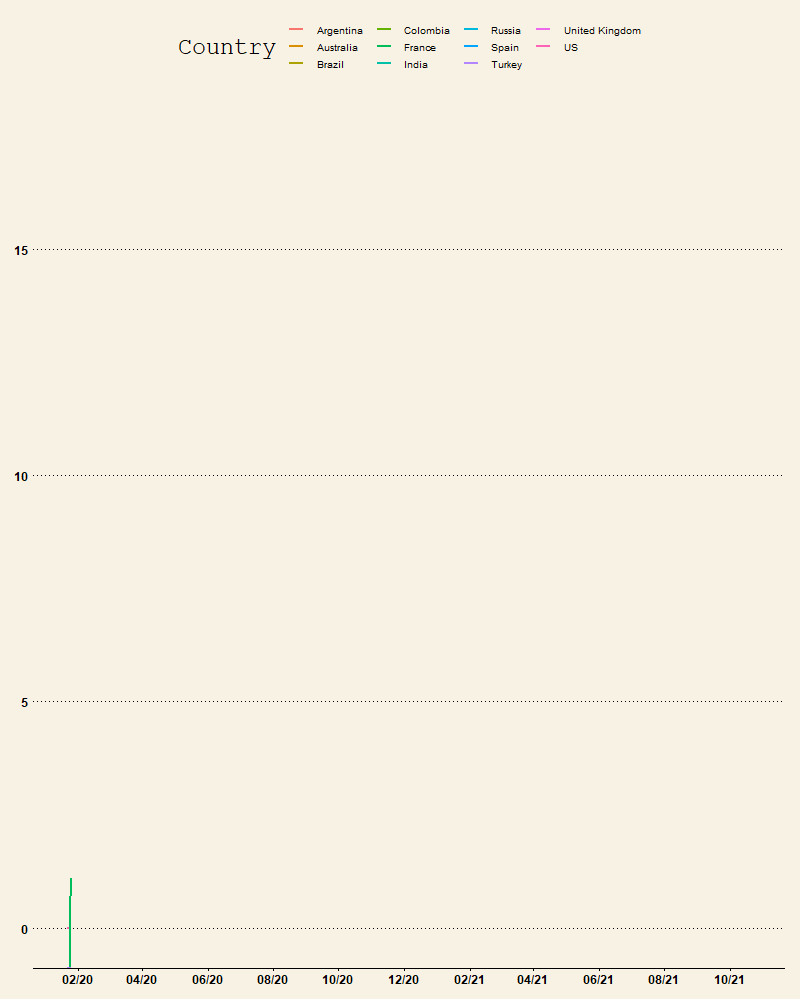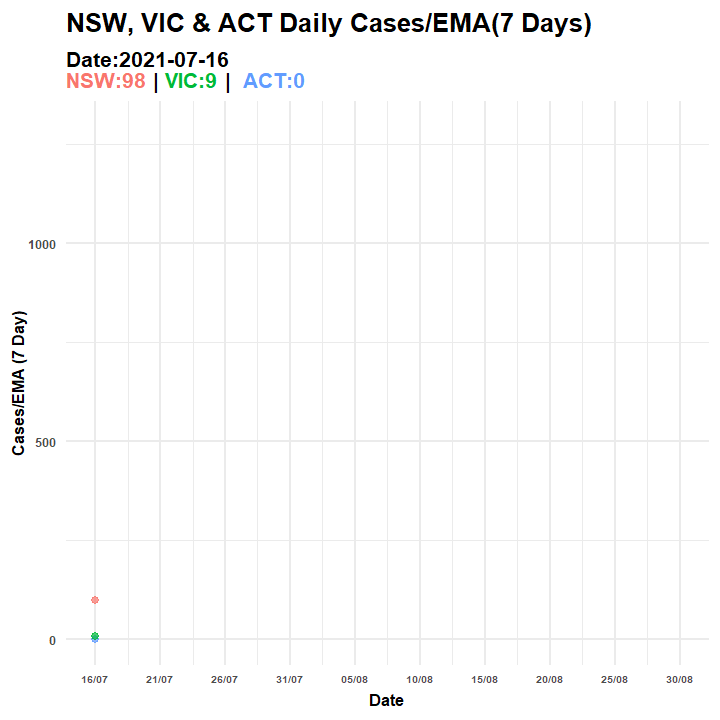## 6.2 Animation using gganimate

• We can also use the gganimate package to convert the image into a gif.
library(gganimate)
p1.anim = p1 + transition_reveal(Date)
anim_p1 = animate(p1.anim, fps = 10, start_pause = 2, end_pause = 5, rewind = FALSE,
width = 800, height = 1000)
anim_save(filename = "covid_cases_log_2021aug.gif", animation = anim_p1)Figure 6.4: Animated Graph

• We can also use further customisations to visualise other information on the plot
• The following example uses data from the 2021 COVID-19 spread in Australia
• The plot shows the number of Covid-19 cases along with the Date in the animation
• The data is the last 3 months data (accessed: 30-08-2021), downloaded from https://www.covid19data.com.au/states-and-territories
• The code below imports the data and selects NSW, VIC and ACT, then creates EMA and SMA using last 7 Days of data.
library(tidyverse)  #using tidyverse here to easily create grouped statistics
# the date column name doesnt look ok so lets rename it
colnames(d_au_3m) = "Date"
# convert to date type
d_au_3m$Date = as.Date(d_au_3m$Date, format = "%d/%m/%y")
d_au_3m = d_au_3m[, c(1:3, 9)]
data_p2 = d_au_3m %>%
pivot_longer(cols = -Date, names_to = "State", values_to = "Cases")
rolling1 = data_p2 %>%
arrange(Date, State, Cases) %>%
group_by(State) %>%
mutate(EMA = TTR::EMA(Cases, n = 7))  #add EMA
rolling1 = rolling1 %>%
arrange(Date, State, Cases) %>%
group_by(State) %>%
mutate(SMA = TTR::SMA(Cases, n = 7))  #add SMA
rolling1 = rolling1[rolling1$Date > as.Date("2021-07-15"), ] #selecting from 15 July 2021 rolling1$State = factor(rolling1$State, levels = c("NSW", "VIC", "ACT")) • Create plots then animate • Plot • The plot first layer has points and lines for the EMA (can replace for SMA or plot both) • The line has an arrow at the end • The scale is changed to display the dates better and some changes are made to theme elements • Animation • A transition_manual is used to access current_frame, cumulative=TRUE keeps the previous data • Title used elements from the ggtext to modify the colors of the group variables • Notice the use of element_markdown() in the ggplot library(ggthemes) library(ggtext) p2 = ggplot(rolling1, aes(Date, Cases)) + geom_point(alpha = 0.7, aes(color = State, group = seq_along(Date), frame = Cases)) + geom_path(aes(y = EMA, color = State), arrow = arrow(ends = "last", type = "closed", length = unit(0.05, "inches")), size = 1.05, show.legend = FALSE) p3 = p2 + scale_x_date(breaks = c(seq(min(rolling1$Date), max(rolling1$Date), by = "5 days")), date_labels = "%d/%m") + scale_color_discrete(name = "") + labs(x = "Date", y = "Cases") + theme_minimal() + theme(title = element_text(face = "bold"), legend.position = "none", axis.title = element_text(size = 8), strip.text.x = element_text(face = "bold"), axis.text.x = element_text(size = 5, face = "bold"), axis.text.y = element_text(size = 6, face = "bold"), legend.title = element_text(size = 10), plot.subtitle = element_markdown()) + labs(x = "Date", y = "Cases/EMA (7 Day)") p2.anim = p3 + transition_manual(Date, cumulative = TRUE) + ggtitle("NSW, VIC & ACT Daily Cases/EMA(7 Days) ", subtitle = "Date:{current_frame}<br><span style='color:#F8766D;'>NSW:{rolling1[rolling1$Date==as.Date(current_frame),3][2,1]} </span> | <span style='color:#00BA38;'>VIC:{rolling1[rolling1$Date==as.Date(current_frame),3][3,1]}</span> | <span style='color:#619CFF;'> ACT:{rolling1[rolling1$Date==as.Date(current_frame),3][1,1]}</span>")

anim_p2 = animate(p2.anim, fps = 8, start_pause = 1, end_pause = 30, detail = 2,
rewind = FALSE, width = 720, height = 720, res = 140, renderer = gifski_renderer())
anim_save(filename = "covid_aus_cases_EMA_aug30_.gif", animation = anim_p2)
`Figure 6.5: Animated Graph (COVID-19 AU)